• 时间序列有高度的自相关性，所以我不能随机打乱。 如果1-6月训练，7、8验证，9、10测试的话，实验结果表明测试精度远差于验证集逻辑上很好解释，中间差了两个月，市场行情发生了很大的变化，模型没见过跟不...机器学习 测试
• ML之FE：特征工程中常用的五大数据集划分方法(特殊类型数据分割，如时间序列数据分割法)讲解及其代码 目录 特殊类型数据分割 5.1、时间序列数据分割TimeSeriesSplit 特殊类型数据分割 5.1、时间序列...
ML之FE：特征工程中常用的五大数据集划分方法(特殊类型数据分割，如时间序列数据分割法)讲解及其代码

目录

特殊类型数据分割

5.1、时间序列数据分割TimeSeriesSplit

特殊类型数据分割

5.1、时间序列数据分割TimeSeriesSplit

class TimeSeriesSplit Found at: sklearn.model_selection._split

class TimeSeriesSplit(_BaseKFold):

"""Time Series cross-validator .. versionadded:: 0.18

Provides train/test indices to split time series data samples that are observed at fixed time intervals, in train/test sets. In each split, test indices must be higher than before, and thus shuffling in cross validator is inappropriate. This cross-validation object is a variation of :class:KFold.  In the kth split, it returns first k folds as train set and the (k+1)th fold as test set.

Note that unlike standard cross-validation methods, successive training sets are supersets of those that come before them.

Read more in the :ref:User Guide <cross_validation>.

Parameters

----------

n_splits : int, default=5. Number of splits. Must be at least 2. .. versionchanged:: 0.22 . n_splits default value changed from 3 to 5.

max_train_size : int, default=None. Maximum size for a single training set.

提供训练/测试索引，以分割时间序列数据样本，在训练/测试集中，在固定的时间间隔观察。在每次分割中，测试索引必须比以前更高，因此在交叉验证器中变换是不合适的。这个交叉验证对象是KFold 的变体。在第k次分割中，它返回第k次折叠作为序列集，返回第(k+1)次折叠作为测试集。

注意，与标准的交叉验证方法不同，连续训练集是之前那些训练集的超集。

更多信息请参见:ref: ' User Guide <cross_validation> '。</cross_validation>

参数

----------

n_splits :int，默认=5。数量的分裂。必须至少是2. ..versionchanged:: 0.22。' ' n_split ' ' '默认值从3更改为5。

max_train_size : int，默认None。单个训练集的最大容量。

Examples

--------

>>> import numpy as np

>>> from sklearn.model_selection import TimeSeriesSplit

>>> X = np.array([[1, 2], [3, 4], [1, 2], [3, 4], [1, 2], [3, 4]])

>>> y = np.array([1, 2, 3, 4, 5, 6])

>>> tscv = TimeSeriesSplit()

>>> print(tscv)

TimeSeriesSplit(max_train_size=None, n_splits=5)

>>> for train_index, test_index in tscv.split(X):

...     print("TRAIN:", train_index, "TEST:", test_index)

...     X_train, X_test = X[train_index], X[test_index]

...     y_train, y_test = y[train_index], y[test_index]

TRAIN:  TEST: 

TRAIN: [0 1] TEST: 

TRAIN: [0 1 2] TEST: 

TRAIN: [0 1 2 3] TEST: 

TRAIN: [0 1 2 3 4] TEST: 

Notes

-----

The training set has size i * n_samples // (n_splits + 1) + n_samples % (n_splits + 1) in the ith split, with a test set of size n_samples//(n_splits + 1), where n_samples is the number of samples.

"""

@_deprecate_positional_args

def __init__(self, n_splits=5, *, max_train_size=None):

super().__init__(n_splits, shuffle=False, random_state=None)

self.max_train_size = max_train_size

def split(self, X, y=None, groups=None):

"""Generate indices to split data into training and test set.

Parameters

----------

X : array-like of shape (n_samples, n_features). Training data, where n_samples is the number of samples and n_features is the number of features.

y : array-like of shape (n_samples,). Always ignored, exists for compatibility.

groups : array-like of shape (n_samples,). Always ignored, exists for compatibility.

Yields

------

train : ndarray. The training set indices for that split.

test : ndarray. The testing set indices for that split.

"""

X, y, groups = indexable(X, y, groups)

n_samples = _num_samples(X)

n_splits = self.n_splits

n_folds = n_splits + 1

if n_folds > n_samples:

raise ValueError(

("Cannot have number of folds ={0} greater than the number of samples: {1}."). format(n_folds, n_samples))

indices = np.arange(n_samples)

test_size = n_samples // n_folds

test_starts = range(test_size + n_samples % n_folds, n_samples,

test_size)

for test_start in test_starts:

if self.max_train_size and self.max_train_size < test_start:

yield indices[test_start - self.max_train_size:test_start], indices

[test_start:test_start + test_size]

else:

yield indices[:test_start], indices[test_start:test_start + test_size]

Examples

--------

>>> import numpy as np

>>> from sklearn.model_selection import TimeSeriesSplit

>>> X = np.array([[1, 2], [3, 4], [1, 2], [3, 4], [1, 2], [3, 4]])

>>> y = np.array([1, 2, 3, 4, 5, 6])

>>> tscv = TimeSeriesSplit()

>>> print(tscv)

TimeSeriesSplit(max_train_size=None, n_splits=5)

>>> for train_index, test_index in tscv.split(X):

...     print("TRAIN:", train_index, "TEST:", test_index)

...     X_train, X_test = X[train_index], X[test_index]

...     y_train, y_test = y[train_index], y[test_index]

TRAIN:  TEST: 

TRAIN: [0 1] TEST: 

TRAIN: [0 1 2] TEST: 

TRAIN: [0 1 2 3] TEST: 

TRAIN: [0 1 2 3 4] TEST: 

Notes

-----

The training set has size i * n_samples // (n_splits + 1) + n_samples % (n_splits + 1) in the ith split, with a test set of size n_samples//(n_splits + 1), where n_samples is the number of samples.

"""

@_deprecate_positional_args

def __init__(self, n_splits=5, *, max_train_size=None):

super().__init__(n_splits, shuffle=False, random_state=None)

self.max_train_size = max_train_size

def split(self, X, y=None, groups=None):

"""Generate indices to split data into training and test set.

Parameters

----------

X : array-like of shape (n_samples, n_features). Training data, where n_samples is the number of samples and n_features is the number of features.

y : array-like of shape (n_samples,). Always ignored, exists for compatibility.

groups : array-like of shape (n_samples,). Always ignored, exists for compatibility.

Yields

------

train : ndarray. The training set indices for that split.

test : ndarray. The testing set indices for that split.

"""

X, y, groups = indexable(X, y, groups)

n_samples = _num_samples(X)

n_splits = self.n_splits

n_folds = n_splits + 1

if n_folds > n_samples:

raise ValueError(

("Cannot have number of folds ={0} greater than the number of samples: {1}."). format(n_folds, n_samples))

indices = np.arange(n_samples)

test_size = n_samples // n_folds

test_starts = range(test_size + n_samples % n_folds, n_samples,

test_size)

for test_start in test_starts:

if self.max_train_size and self.max_train_size < test_start:

yield indices[test_start - self.max_train_size:test_start], indices

[test_start:test_start + test_size]

else:

yield indices[:test_start], indices[test_start:test_start + test_size]


展开全文• 数据进行活动窗口均值处理，根据其均值进行数据分类，具有时间连贯性。matlab代码
• 针对模糊时间序列研究中比率划分论域方法存在对非均匀数据划分效果不理想的缺陷, 提出一种基于模糊<em>C</em> 均值聚类(FCM) 算法的多尺度比率划分论域的方法. 首先利用FCM算法对样本数据进行分类; 然后计算各类数据...
• ML之FE：特征工程中常用的五大数据集划分方法(留1法/留p法、随机划分法、K折交叉验证法、自定义分割法、时间序列数据分割法)讲解及其代码实现 目录 特征工程中常用的数据集划分方法 1、留一法、留P法 T1、留...
ML之FE：特征工程中常用的五大数据集划分方法(留1法/留p法、随机划分法、K折交叉验证法、自定义分割法、时间序列数据分割法)讲解及其代码实现

目录

特征工程中常用的数据集划分方法

1、留一法、留P法

T1、留一法 LeaveOneOut

T2、留P法 LeavePOut

2、随机划分法

T1、全部数据随机使用

T2、全部数据按比例随机使用

T3、类分布随机使用

3、K折交叉验证

T1、KFold 用法：不同子集可部分相交

T2、GroupKFold 用法：不同子集不相交

T3、StratifiedKFold 用法：保证训练集中每一类的比例是相同的

特征工程中常用的数据集划分方法

1、留一法、留P法

根据label_n类别个数，有放回的自动进行label_n次切分，每次留下1个或P个作为验证集

T1、留一法 LeaveOneOut


import numpy as np
X=np.array([[11,12,13,14,15,16],[21,22,23,24,25,26],[31,32,33,34,35,36],[41,42,43,44,45,46],[51,52,53,54,55,56],[61,62,63,64,65,66]])
y=np.array([1,2,3,4,5,6])

#1、留一法、留P法：根据label_n类别个数，有放回的自动进行label_n次切分，每次留下1个或P个作为验证集

#T1、留一法 LeaveOneOut：
from sklearn.model_selection import LeaveOneOut
#LeaveOneOut 使用方法
select_LOO=LeaveOneOut()
select_LOO.get_n_splits(X)
print('LeaveOneOut 用法：',select_LOO)
#LeaveOneOut 做了什么：输出 LeaveOneOut 实现的内容
for train_index,test_index in select_LOO.split(X,y):
print("Train Index:",train_index,",Test Index:",test_index)
X_train,X_test=X[train_index],X[test_index]
y_train,y_test=y[train_index],y[test_index]
print('X_train,X_test：\n',X_train,X_test)
print('y_train,y_test：\n',y_train,y_test)


T2、留P法 LeavePOut

测试集留下P个


#T2、留P法 LeavePOut ：测试集留下P个
from sklearn.model_selection import LeavePOut

select_LPO=LeavePOut(p=2)
select_LPO.get_n_splits(X)
print('LeavePOut 用法：',select_LPO)
for train_index,test_index in select_LPO.split(X,y):
print("Train Index:",train_index,",Test Index:",test_index)
X_train,X_test=X[train_index],X[test_index]
y_train,y_test=y[train_index],y[test_index]
print('X_train,X_test：\n',X_train,X_test)
print('y_train,y_test：\n',y_train,y_test)


2、随机划分法

均需先把原数据打乱再选择，即保证随机性。

T1、全部数据随机使用

test_size取所有训练数据的25%=6*0.25≈2个样例，没有指定train_size意味着取剩下的全部6-2=4个样例

#2、随机划分法：均需先把原数据打乱再选择，即保证随机性
from sklearn.model_selection import ShuffleSplit

#T1、全部数据随机使用：：test_size取所有训练数据的25%=6*0.25≈2个样例，没有指定train_size意味着取剩下的全部6-2=4个样例
select_SS=ShuffleSplit(n_splits=3,test_size=.25,random_state=0)
select_SS.get_n_splits(X)
print('ShuffleSplit 用法：',select_SS)
for train_index,test_index in select_SS.split(X,y):
print("Train Index:",train_index,",Test Index:",test_index)
X_train,X_test=X[train_index],X[test_index]
y_train,y_test=y[train_index],y[test_index]
#     print('X_train,X_test：\n',X_train,X_test)
#     print('y_train,y_test：\n',y_train,y_test)


T2、全部数据按比例随机使用

train_size取所有训练数据的50%=6*0.5≈3个样例，test_size取所有训练数据的25%=6*0.25≈2个样例

#T2、全部数据按比例随机使用：train_size取所有训练数据的50%=6*0.5≈3个样例，test_size取所有训练数据的25%=6*0.25≈2个样例
select_SS02=ShuffleSplit(n_splits=3,train_size=.5,test_size=.25,random_state=0)
select_SS02.get_n_splits(X)
print('select_SS02 用法：',select_SS02)
for train_index,test_index in select_SS02.split(X,y):
print("Train Index:",train_index,",Test Index:",test_index)



T3、类分布随机使用

训练集和测试集的比例随机选定，训练集和测试集的比例的和可以小于1,但是还要保证训练集中各类所占的比例是一样的

#T3、类分布随机使用：训练集和测试集的比例随机选定，训练集和测试集的比例的和可以小于1,但是还要保证训练集中各类所占的比例是一样的
from sklearn.model_selection import StratifiedShuffleSplit
y=np.array([123,123,123,123,456,456])

select_SSS=StratifiedShuffleSplit(n_splits=3,test_size=.5,random_state=0)
select_SSS.get_n_splits(X,y)
print('StratifiedShuffleSplit 用法：',select_SSS)
for train_index,test_index in select_SSS.split(X,y):
print("Train Index:",train_index,",Test Index:",test_index)
X_train,X_test=X[train_index],X[test_index]
y_train,y_test=y[train_index],y[test_index]
print('X_train,X_test：\n',X_train,X_test)
print('y_train,y_test：\n',y_train,y_test)


3、K折交叉验证

KFold，GroupKFold，StratifiedKFold

T1、KFold 用法：不同子集可部分相交

#3、K折交叉验证：KFold，GroupKFold，StratifiedKFold

#T1、KFold 用法：不同子集可部分相交
from sklearn.model_selection import KFold

#KFold 使用方法
select_KFold=KFold(n_splits=4)    #分成n_splits个组。比如共有6个样本，分成3组，即3个不相交的子集。
select_KFold.get_n_splits(X)
print('KFold 用法：',select_KFold)

#KFold 做了什么：输出 KFold 实现的内容
for train_index,test_index in select_KFold.split(X):
print("Train Index:",train_index,",Test Index:",test_index)
X_train,X_test=X[train_index],X[test_index]
y_train,y_test=y[train_index],y[test_index]
print('X_train,X_test：\n',X_train,X_test)
print('y_train,y_test：\n',y_train,y_test)



T2、GroupKFold 用法：不同子集不相交

#T2、GroupKFold 用法：不同子集不相交
import numpy as np
from sklearn.model_selection import GroupKFold

#GroupKFold 使用方法
groups=np.array([1,2,3,4,5,6])
select_GKFold=GroupKFold(n_splits=2)
select_GKFold.get_n_splits(X,y,groups)
print('GroupKFold 用法：',select_GKFold)

#GroupKFold 做了什么：输出GroupKFold实现的内容
for train_index,test_index in select_GKFold.split(X,y,groups):
print("Train Index:",train_index,",Test Index:",test_index)
X_train,X_test=X[train_index],X[test_index]
y_train,y_test=y[train_index],y[test_index]
print('X_train,X_test：\n',X_train,X_test)
print('y_train,y_test：\n',y_train,y_test)



T3、StratifiedKFold 用法：保证训练集中每一类的比例是相同的

#T3、StratifiedKFold 用法：保证训练集中每一类的比例是相同的
import numpy as np
from sklearn.model_selection import StratifiedKFold
y=np.array([123,123,123,456,456,456])
select_SKFold=StratifiedKFold(n_splits=3)
select_SKFold.get_n_splits(X,y)
print('StratifiedKFold 用法：',select_SKFold)
for train_index,test_index in select_SKFold.split(X,y):
print("Train Index:",train_index,",Test Index:",test_index)
X_train,X_test=X[train_index],X[test_index]
y_train,y_test=y[train_index],y[test_index]
print('X_train,X_test：\n',X_train,X_test)
print('y_train,y_test：\n',y_train,y_test)




展开全文• 时间序列划分训练集与测试集问题 问题： 使用sklearn的train_test_split进行训练集和测试集数据划分后，数据的顺序被打乱，不再按照日期排序。这时候预测的准确度高达80%。鉴于这个准确度比较吓人，我有点怀疑。于是...
时间序列划分训练集与测试集问题
问题：
使用sklearn的train_test_split进行训练集和测试集数据划分后，数据的顺序被打乱，不再按照日期排序。这时候预测的准确度高达80%。鉴于这个准确度比较吓人，我有点怀疑。于是就对数据按照日期顺序划分训练集和测试集，比如2015年之前的作为训练集，之后的作为测试集。重新训练后，预测准确度下降到了50%。
知乎的回答


展开全文• 通过 Python 代码实现时间序列数据的统计学预测模型 在本篇中，我们将展式使用 Python 统计学模型进行时间序列数据分析。 *** 问题描述 *** 目标：根据两年以上的每日广告支出历史数据，提前预测两个月的广告支出...
通过 Python 代码实现时间序列数据的统计学预测模型
在本篇中，我们将展式使用 Python 统计学模型进行时间序列数据分析。
*** 问题描述 ***
目标：根据两年以上的每日广告支出历史数据，提前预测两个月的广告支出金额。
原始数据：2017-01-01 到 2019-09-23 期间的每日广告支出。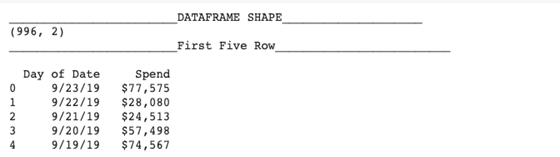数据准备：划分训练集和测试集。
df1 = data[['Date','Spend']].set_index('Date')
train = df1.iloc[:933,:]
test = df1.iloc[933:,:]
test.shape,train.shape

测试集大小：(63,1)；训练集大小：(933,1)。
本文目录
综述
时间序列分析常用统计模型
单变量时间序列数据建模的关键要素
ARIMA
ACF 和 PACF
SARIMA
案例：通过 SARIMA 预测广告支出
ETS
ETS
Holt-Winter 季节性预测算法
案例：通过 Holt-Winter 季节性预测算法预测广告支出
算法对比
结束语
综述
时间序列分析常用统计模型
单变量时间序列统计学模型，如：平均方法、平滑方法、有/无季节性条件的 ARIMA 模型。
多变量时间序列统计学模型，如：外生回归变量、VAR。
附加或组件模型，如：Facebook Prophet、ETS。
结构化时间序列模型，如：贝叶斯结构化时间序列模型、分层时间序列模型。
在本篇文章中，我们主要关注 SARIMA 和 Holt-winters 方法。
单变量时间序列统计学模型的关键要素
如果我们想要对时间序列数据进行上述统计学模型分析，需要进行一系列处理使得：(1)数据均值 (2)数据方差 (3)数据自协方差 这三个指标不依赖于时间项。即时间序列数据具有平稳性。
如何明确时间序列数据是否具有平稳性？可以从两个特征进行判断。(1) 趋势，即均值随时间变化；(2) 季节性，即方差随时间变化、自协方差随时间变化。若满足以上两个条件，则时间序列数据不符合平稳性要求。
可以通过以下方法消除上述问题：
变换，如：取对数、取平方等。
平滑处理，如：移动平均等。
差分。
分解。
多项式拟合，如：拟合回归。
ARIMA
Autoregressive Integrated Moving Average model (ARIMA)，差分整合移动平均自回归模型。ARIMA(p,d,q)主要包含三项：
p:AR项，即自回归项(autoregression)，将时间序列下一阶段描述为前一阶段数据的线性映射。
d项，即积分项(integration)，时间序列的差分预处理步骤，使其满足平稳性要求
q:MA项，即移动平均项(moving average)，将时间序列下一阶段描述为前一阶段数据平均过程中的残留误差的线性映射。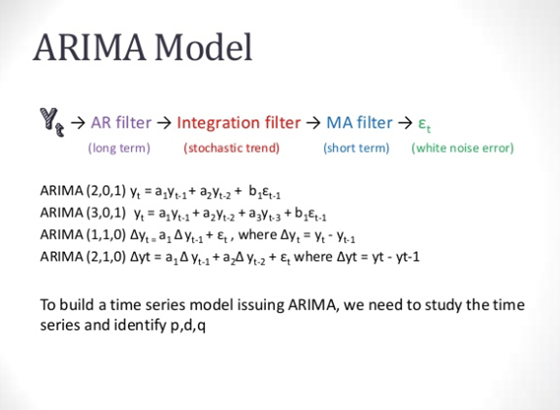该模型需要指定 p d q 三项参数，并按照顺序执行。ARIMA 模型也可以用于开发 AR, MA 和 ARMA 模型。
ACF 和 PACF 图
自相关函数，autocorrelation function(ACF)，描述了时间序列数据与其之后版本的相关性（如：Y(t) 与 Y(t-1) 之间的相关性）。
偏自相关函数，partial autocorrelation function(PACF)，描述了各个序列的相关性。
通过 PACF 图可以确定 p
通过 ACF 图可以确定 q
SARIMA
季节性差分自回归滑动平均模型，seasonal autoregressive integrated moving averaging(SARIMA)，在 ARIMA 模型的基础上进行了季节性调节。
其形式为：SARIMA(p,d,q)(P,D,Q)s，其中P,D,Q为季节参数，s为时间序列周期。
案例：通过 SARIMA 预测广告支出
首先，我们建立 test_stationarity 来检查时间序列数据的平稳性。
from statsmodels.tsa.stattools import adfuller
df1=df.resample('D', how=np.mean)

def test_stationarity(timeseries):
rolmean = timeseries.rolling(window=30).mean()
rolstd = timeseries.rolling(window=30).std()

plt.figure(figsize=(14,5))
sns.despine(left=True)
orig = plt.plot(timeseries, color='blue',label='Original')
mean = plt.plot(rolmean, color='red', label='Rolling Mean')
std = plt.plot(rolstd, color='black', label = 'Rolling Std')

plt.legend(loc='best'); plt.title('Rolling Mean & Standard Deviation')
plt.show()

print ('<Results of Dickey-Fuller Test>')
dftest = adfuller(timeseries, autolag='AIC')
dfoutput = pd.Series(dftest[0:4],     index=['Test Statistic','p-value','#Lags Used','Number of Observations Used'])
for key,value in dftest.items():
dfoutput['Critical Value (%s)'%key] = value
print(dfoutput)
test_stationarity(df1.Spend.dropna())

通过 test_stationarity 函数，可以绘制移动平均值以及标准差，并且通过 Augmented Dickey-Fuller test 输出 P 值。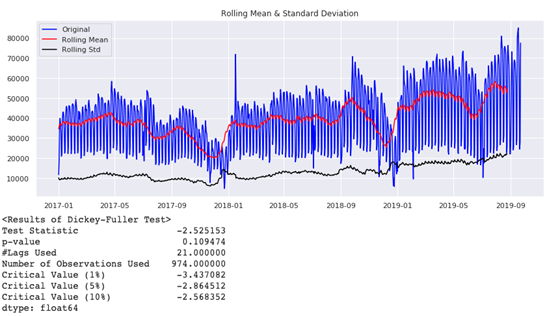对比临界值(critical value)可以看到，时间序列数据时非平稳的。
首先我们试试对数变换，能不能使数据达到平稳性要求。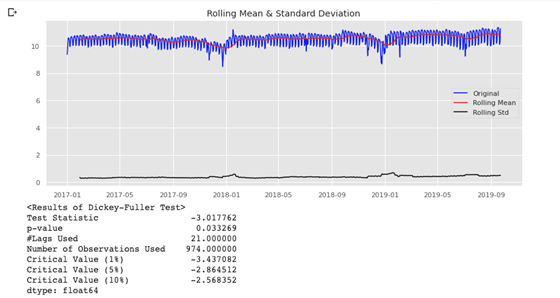可以看到，利用对数变换df1[‘log_Spend’]=np.log(df1[‘Spend’])，时间序列在阈值为5%时满足平稳性要求。
接下来，我们试试差分操作：test_stationarity(df1[‘Spend’].diff(1).dropna())。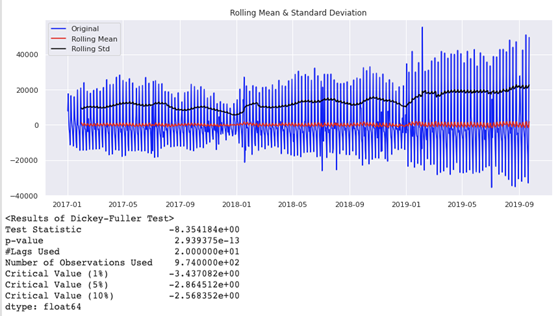显然，通过差分操作后，效果更好，时间序列在阈值为1%时满足平稳性要求。
然后，我们就可以建立 SARIMA 模型，并且预测 2019-07-23 到 2019-09-23 这两个月间每天的广告指出。
import statsmodels.api as sm
fit1 = sm.tsa.statespace.SARIMAX(train.Spend, order=(7, 1, 2), seasonal_order=(0, 1, 2, 7)).fit(use_boxcox=True)
test['SARIMA'] = fit1.predict(start="2019-07-23", end="2019-09-23", dynamic=True)
plt.figure(figsize=(16, 8))
plt.plot(train['Spend'], label='Train')
plt.plot(test['Spend'], label='Test')
plt.plot(test['SARIMA'], label='SARIMA')
plt.legend(loc='best')
plt.show()

现在，让我们通过从 sklearn.metrics 包导入 mean_squared_error，mean_absolute_error 函数计算 mse 和 mae 来检查这个模型的性能。结果如下：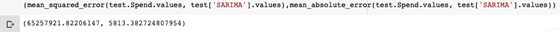进行数据可视化：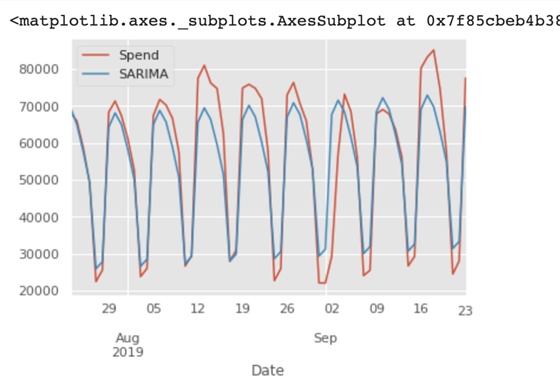从 mse、mae 以及预测曲线可以看出，SARIMA 模型成功预测了时间序列变化趋势和季节性特征。但是在峰值处的表现仍旧有待提高。
ETS
ETS，Exponential Smoothing
由于时间序列数据随时间变化但具有一定的随机性，我们通常希望对数据进行平滑处理。为此，我们将使用 ETS 技术，通过指数方法为过去的数据分配较少的权重。同时将时间序列数据分解为趋势（T）、季节（S）和误差（E）分量。
三种常用 ETS 方法如下：
Linear：双指数平滑；
Additive：三指数平滑；
Multiplicative：三指数平滑。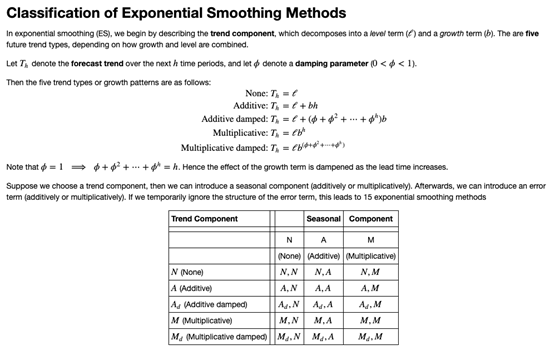Holt-Winter 季节性预测算法
Holt-winter 季节性预测算法是一种三指数平滑方法。它包含三个主要部分：水平、趋势、季节性分量。
案例：通过 Holt-Winter 季节性预测算法预测广告支出
通过 Holt-winter 季节性预测算法预测 2019-07-23 到 2019-09-23 期间的每日广告支出，代码如下：
from statsmodels.tsa.api import ExponentialSmoothing
fit1 = ExponentialSmoothing(np.asarray(train['Spend']) ,seasonal_periods=7 ,trend='add', seasonal='add').fit(use_boxcox=True)
test['Holt_Winter'] = fit1.forecast(len(test))
plt.figure(figsize=(16,8))
plt.plot( train['Spend'], label='Train')
plt.plot(test['Spend'], label='Test')
plt.plot(test['Holt_Winter'], label='Holt_Winter')
plt.legend(loc='best')
plt.show()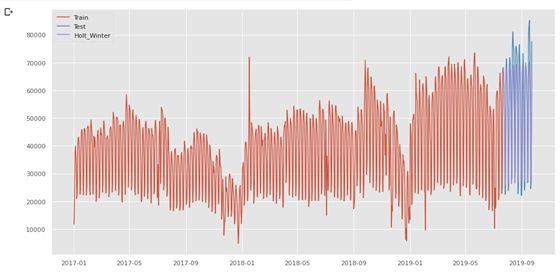同样，我们通过 mean_squared_error,mean_absolute_error 函数查看 mse 和 mae。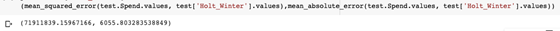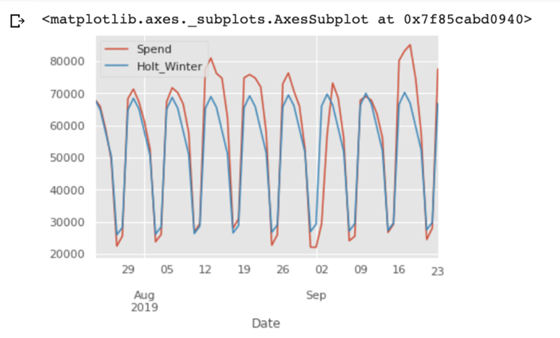可以看到，H-W 模型同样能够预测时间序列变化趋势和季节性特征。
算法对比
通过将两种算法的预测结果进行对比，可以评价哪种方法预测能力更好。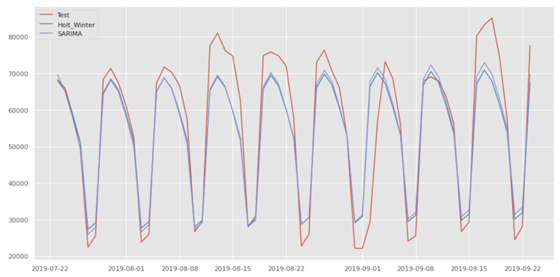从图中可以看出，在MSE和MAE均较低的情况下，SARIMA模型的性能略优于Holt-Winter模型。尽管这两种模式都无法完美地抓住峰谷特征，但它们仍然对企业有用。根据数据，平均每月广告支出为2百万美元以上。而这两种算法的MAE大约在6000左右。换言之，对于一家平均每月广告支出为2百万美元的企业，两个月的广告支出预测误差只在6000美元左右，这是相当可观的。
结束语
在本文中，单变量预测方法在广告支出数据上表现良好。但这些方法难以组合/合并新的信号（如事件、天气）。同时这些方法对丢失数据也非常敏感，通常不能很好地预测很长一段时间。
而在未来的文章中，我们将展示如何使用深度学习技术来预测同一数据集上的时间序列！展开全文• 有时候我们的数据是按某个频率收集的，比如每日、每月、每15分钟，那么我们怎么产生对应频率的索引呢？pandas中的date_range可用于生成指定长度的DatetimeIndex。我们先看一下怎么生成日期范围：pd.date_range...
• 我想请教下有哪些简便的易于操作的一维时间序列聚类算法呢，简单的指定聚类数目（例如：2类），做一下划分就行。 数据是带序号的，不是下面图1这种聚类，而是图2这种...神经网络 python 开发语言 r语言
• 在本篇中，我们将展示使用 Python 统计学模型进行时间序列数据分析。 问题描述 目标：根据两年以上的每日广告支出历史数据，提前预测两个月的广告支出金额。 原始数据：2017-01-01 到 2019-09-23 期间的每日广告支出...python
• 在本篇中，我们将展式使用 Python 统计学模型进行时间序列数据分析。 问题描述目标：根据两年以上的每日广告支出历史数据，提前预测两个月的广告支出金额。 原始数据：2017-01-01 到 2019-09-23 期间的每日广告...
• 在对具有高噪声和非线性的时间序列进行长期预测的研究中，序列数据的趋势信息和波动范围通常比时间点的特定值的预测更有价值和实用。 针对模糊信息颗粒（图）的可变长度分区，构造了一种称为逐步线性除法（SLD）的...研究论文
• 机器学习中对于时间序列数据进行train、val、test划分时需要注意的问题是，不要将val中的数据出现在train中，准确的说，是选取的val数据的时间节点不能与train中数据时间节点有任何交集。否则，你会发现训练时，...机器学习
• 想对时间序列进行状态划分时间序列最大值1.8，最小值-0.5，划分为115个状态区间，每个状态区间宽度为0.02，把处于各状态区间的值化成区间中间的值，写了一段代码，但是得到的值有的小数位数很奇怪，请大神帮忙解惑...
• 数据挖掘中 往往都会遇到时间序列问题，很多比赛或者项目都要对时间进行分组。但是面对众多保存类型，怎么才能避免数据类型错误，提取到想要的时间块呢，现在针对多种情况说一下。我们来看这个数据中time项。是一个...
• 时间序列聚类：无监督，旨在将未标记的时间序列对象划分为同质的组/集群。同一集群中的时间序列比其他集群中的时间序列彼此更相似 算法功能： 识别整个序列的联合动态 消除序列之间的滞后（时移）（通常称为滞后...机器学习
• 已知完成每个组件的开发时间保存在一个数组A里面，每个工程师只能独立完成连续的组件， 并且所有的工程师都是并行工作， 请输出完成这个软件需要的最少时间 输入说明 程序从当前路径下的data.txt文件中读取测试...
• 想对时间序列进行状态划分时间序列最大值1.8，最小值-0.5，划分为115个状态区间，每个状态区间宽度为0.02，把处于各状态区间的值化成区间中间的值，写了一段代码，但是得到的值有的小数位数很奇怪，请大神帮忙解惑...
• 问 题想对时间序列进行状态划分时间序列最大值1.8，最小值-0.5，划分为115个状态区间，每个状态区间宽度为0.02，把处于各状态区间的值化成区间中间的值，写了一段代码，但是得到的值有的小数位数很奇怪，请大神...
•        ...时间序列预处理流程图（侵删） 下面来详细介绍每个阶段的处理 数据预处理流程图 数据预处理-平稳性检验机器学习 人工智能
• 该算法首先对时间序列数据进行向量形式转换，计算出各个时间序列间的相似度并构建复杂网络，然后利用基于Normal矩阵的方法进行复杂网络社团划分，同一类的时间序列被划分到一个社团，即实现对时间序列数据的聚类。...复杂网络
• 针对模糊时间序列对于预测不...虑数据点固有的模糊不确定性, 较好地反映了系统的特征分布, 提高了复杂环境中时间序列的预测性能且允许处理 多因子预测问题. 最后通过实例验证了所提出方法的有效性和优越性.</p>
• 为了实现时间序列自动聚类,以及更为细致地描述时间序列之间的结构关系,引入社区发现...实验结果表明,所提方法无需确定初始簇中心,能够有效划分人工数据网络和真实社会网络,在时间序列数据聚类中取得了良好的聚类效果.
• ts.data=ts(data, frequency=12,start =2016)#将数据划分时间序列数据（按照月份）需要注意的是，data是单变量数据 train=window（ts.data,start=2016,end =2016+11/12) test=window(ts.dar语言
• 我正在处理来自netCDF文件的气候数据。来自不同模型的数据具有不同的分辨率-因此，有必要将模型“重新划分”为一个共同的网格分辨率。数据是三维的(时间、纬度、经度)。...在有没有什么方法可以有效地在时间序列的...
• 我有一个高速公路上的车速数据集。...另外，这是自相关图(ACF)以上两个图的可视化使我们想到了季节性因素，因此，一个非平稳时间序列，这对我来说毫无疑问。在然而，为了确保非平稳性，我对其进行了Dickey-Ful...
• 上篇说到了数据预处理和EDA，数据预处理是为了...对于我们这个任务而言，由于是时间序列问题，很多地方和其他类问题的处理不一样，比如时间序列问题在构造特征的时候就要包括到历史特征，还有时间窗特征，包括时间窗...特征工程 kaggle
• 在充分利用时间序列时变特征的基础上，...该方法在有效地提取序列中的趋势和压缩原始数据的同时，能够随着时间序列长度的增长对序列进行划分，具有高效、实现方法简便、效果直观的优点，对于不同领域的数据适应性良好。分段线性表示
• ，其中包含85个单变量时间序列数据集。 ，其中包含13个多元时间序列数据集。 码 代码划分如下： python文件包含运行实验所需的代码。 文件夹包含必要的功能，以读取数据集并可视化绘图。 文件夹包含9个python...
• 基于matlab2019a编写的程序 深度置信网络时间序列预测，已经调好的程序，换了数据就可以用。无标签训练 划分训练集和测试集...